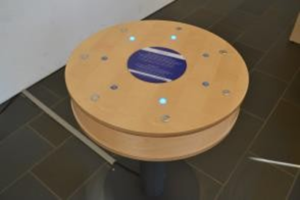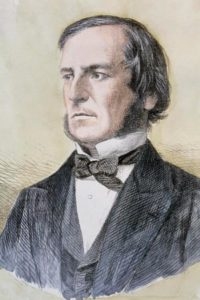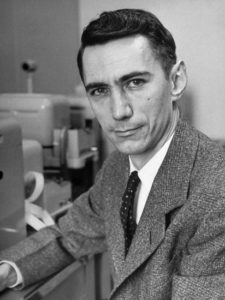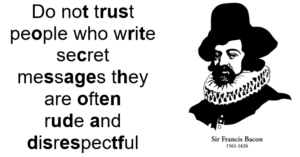# Lights OnExperiment with the buttons. What do they do? Can you turn all lights on? This can always be done in seven or fewer moves. Does the order you press the buttons matter? Can you describe which states are one move away from solved? Which states are two moves away from solved?
Maths

Each light can be either “on” or “off”. If a light is on, label it with a 1. If a light is off, label it with 0. There are seven lights, so if all lights are off the board is labelled 0000000, if all lights are on the board is labelled 1111111. All other states are a mixture of 1s and 0s.
Mathematically, pressing the first button is the same as adding the number 1110000 to the board state, with the special rule that 1 + 1 = 0. This is called XOR addition.
The problem then becomes, starting with board state 0000000 (or some other board state), can we achieve board state 1111111, by adding some combination of the numbers 1110000, 0111000, 0011100, 0001110, 0000111.
Like regular addition, order does not matter, so if you find one solution, pressing the buttons in a different order will also work. XOR addition is special because addition and subtraction are the same, adding 1110000 is the same as subtracting 1110000.

History

There are several counting or writing systems that use only two symbols. For example, Morse code uses dots and dashes, while braille uses raised bumps. Binary is a systematic way of writing numbers using 1s and 0s. It was British mathematician George Boole who first started studying ideas in logic using 1s and 0s, with the strange idea that 1+1=0. These ideas were later used by American mathematician Claude Shannon when designing electronic relays, which laid the foundation for digital circuits that can be found in all modern computers.

PeopleGeorge Boole 1815-1864 George Boole was an English mathematician who was largely self-taught. His work on logic is the foundation of modern mathematical logic and the information age. Claude Shannon 1916-2001 Claude Shannon was an American mathematician and electrical engineer. His work on electronic relays is the foundation of modern computer circuits. Shannon is also the father of modern digital communication, as he explored how we can send messages using 1s and 0s, while being efficient, reliable and secretive.Applications

Binary numbers, Boolean algebra and XOR addition are the foundations of modern computer circuits and communication. For example, any message can be transmitted as a sequence of 1s and 0s. Your computer can then generate a random (or pseudo-random) string of 1s and 0s and add this sequence to your original message using XOR addition. The result is an unbreakable (or near unbreakable) code.

Maths at Home

Binary codes are a way to send messages using two symbols only. The first binary code was the Bacon cipher – the tastiest of all the ciphers.

The Bacon cipher allows you to hide messages in plain sight using two different fonts.

Replace letters in bold with 1, and all other letters with 0. Then each group of five letters is a binary number. If space = 00000, A = 00001, B = 00010, C = 00011 etc, what is the hidden message below?The Bacon cipher was invented by the scientist Sir Francis Bacon as a way to send secret messages. In the early days of the printing press it was common to see things printed in two fonts.